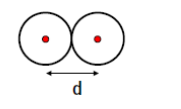# Problem: A diatomic molecule such as molecular nitrogen (N2) consists of two atoms each of mass M, whose nuclei are a distance d apart. What is the moment of inertia of the molecule about its center of mass?A. M d 2B. 2M d 2C. 4M d 2D. 1/2 M d 2 E. 1/4 M d 2

###### FREE Expert Solution
89% (460 ratings)
###### Problem Details

A diatomic molecule such as molecular nitrogen (N2) consists of two atoms each of mass M, whose nuclei are a distance d apart. What is the moment of inertia of the molecule about its center of mass?

A. M d 2

B. 2M d 2

C. 4M d 2

D. 1/2 M d 2

E. 1/4 M d 2